# Arc and segment

Calculate the length of circular arc l, area of the circular arc S1 and area of circular segment S2. Radius of the circle is 11 and corresponding angle is
$\dfrac{ 2}{ 12} \pi$
.

Correct result:

l =  5.8
S1 =  31.7
S2 =  1.4

#### Solution:

$l = \dfrac{ 2}{ 12} \pi \cdot 11 = 5.8$
$S_1 = \dfrac{ 2}{ 2 \cdot 12} \pi \cdot 11^2 = 31.7$
$S_2 = \dfrac{ 1}{ 2 } \cdot 11^2 ( \dfrac{ 2}{ 12} \pi - \sin \dfrac{ 2}{ 12} \pi ) = 1.4$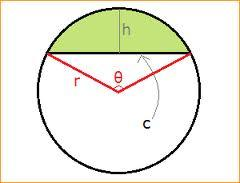Our examples were largely sent or created by pupils and students themselves. Therefore, we would be pleased if you could send us any errors you found, spelling mistakes, or rephasing the example. Thank you!

Please write to us with your comment on the math problem or ask something. Thank you for helping each other - students, teachers, parents, and problem authors.Tips to related online calculators
Most natural application of trigonometry and trigonometric functions is a calculation of the triangles. Common and less common calculations of different types of triangles offers our triangle calculator. Word trigonometry comes from Greek and literally means triangle calculation.

#### You need to know the following knowledge to solve this word math problem:

We encourage you to watch this tutorial video on this math problem:

## Next similar math problems:

• Calculate 6Calculate the distance of a point A[0, 2] from a line passing through points B[9, 5] and C[1, -1].
• Four prismsQuestion No. 1: The prism has the dimensions a = 2.5 cm, b = 100 mm, c = 12 cm. What is its volume? a) 3000 cm2 b) 300 cm2 c) 3000 cm3 d) 300 cm3 Question No.2: The base of the prism is a rhombus with a side length of 30 cm and a height of 27 cm. The heig
• Truncated cone 6Calculate the volume of the truncated cone whose bases consist of an inscribed circle and a circle circumscribed to the opposite sides of the cube with the edge length a=1.
• Edge of prismThe regular quadrilateral prism has a surface of 250 dm2, its shell has a content of 200 dm2. Calculate its leading edge.
• The funnel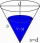The funnel has the shape of an equilateral cone. Calculate the content of the area wetted with water if you pour 3 liters of water into the funnel.
• Chord of triangle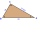If the whole chord of the triangle is 14.4 cm long, how do you calculate the shorter and longer part?
• Integer sidesA right triangle with an integer length of two sides has one leg √11 long. How much is its longest side?
• SailboatThe 20 m long sailboat has an 8 m high mast in the middle of the deck. The top of the mast is fixed to the bow and stern with a steel cable. Determine how much cable is needed to secure the mast and what angle the cable will make with the ship's deck.
• Cylinder containerThe cylindrical container with a diameter of 1.8 m contains 2,000 liters of water. How high does the water reach?
• Hexagonal pyramidFind the volume of a regular hexagonal pyramid, the base edge of which is 12 cm long and the side edge 20 cm.
• Equilateral coneWe pour so much water into a container that has the shape of an equilateral cone, the base of which has a radius r = 6 cm, that one-third of the volume of the cone is filled. How high will the water reach if we turn the cone upside down?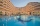Up to 48 rooms, some of which are triple and some quadruple, accommodated 173 people so that all beds are occupied. How many triple and how many quadruple rooms were there?X=2, y=-5 and z=3 what is the value of x-2y?The sum of two numbers is 12. Find these numbers if: a) The sum of their third powers is minimal. b) The product of one with the cube of the other is maximal. c) Both are positive and the product of one with the other power of the other is maximal.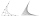In the equation 2x ^ 2 + bx-9 = 0 is one root x1 = -3/2. Determine the second root and the coefficient b.From the window of the building at a height of 7.5 m, the top of the factory chimney can be seen at an altitude angle of 76° 30 ′. The base of the chimney can be seen from the same place at a depth angle of 5° 50 ′. How tall is the chimney?The money - coins are minted from the hardest bronze, which contains copper and tin in a ratio of 41: 9. How much copper and tin are in 2kg of bronze money?